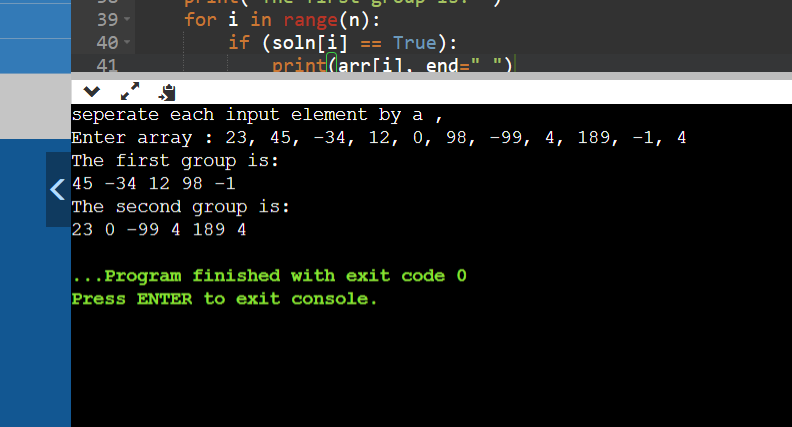## Tug Of War Problem Statement :

Given a set of n integers, divide the set in two subsets of n/2 sizes each such that the difference of the sum of two subsets is as minimum as possible. If n is even, then sizes of two subsets must be strictly n/2 and if n is odd, then size of one subset must be (n-1)/2 and size of other subset must be (n+1)/2.

## Example :

Given input set of integers : {3, 4, 5, -3, 100, 1, 89, 54, 23, 20}

Here size of given array is 10 so output should be two sets of length 5.

If set 1 consists elements : {45, -34, 12, 98, -1} and

set 2 consists elements : {3, 5, -3, 89, 54}

Then sum of elements of both set 1 and aet 2 will be 148

## Code Solution :

```				```
def tugOfWar(arr, n, curr_elements, no_of_selected_elements, soln, min_diff, Sum, curr_sum, curr_position):
if (curr_position == n):
return

if ((int(n / 2) - no_of_selected_elements) > (n - curr_position)):
return

tugOfWar(arr, n, curr_elements, no_of_selected_elements, soln, min_diff, Sum, curr_sum, curr_position + 1)

no_of_selected_elements += 1
curr_sum = curr_sum + arr[curr_position]
curr_elements[curr_position] = True

if (no_of_selected_elements == int(n / 2)):
if (abs(int(Sum / 2) - curr_sum) < min_diff):
min_diff = abs(int(Sum / 2) - curr_sum)
for i in range(n):
soln[i] = curr_elements[i]
else:
tugOfWar(arr, n, curr_elements, no_of_selected_elements, soln, min_diff, Sum, curr_sum, curr_position + 1)

curr_elements[curr_position] = False

def tugOfWar1(arr, n):
curr_elements = [None] * n
soln = [None] * n

min_diff = 

Sum = 0
for i in range(n):
Sum += arr[i]
curr_elements[i] = soln[i] = False

tugOfWar(arr, n, curr_elements, 0, soln, min_diff, Sum, 0, 0)

print("The first group is: ")
for i in range(n):
if (soln[i] == True):
print(arr[i], end=" ")
print()
print("The second group is: ")
for i in range(n):
if (soln[i] == False):
print(arr[i], end=" ")

print("seperate each input element by a ,")
arr = list(map(int,input("Enter array : ").strip().split(",")))
n = len(arr)
tugOfWar1(arr, n)
```
```